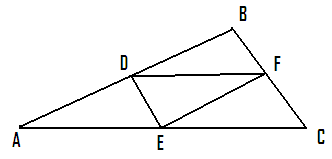7.2 Distance between two points and Section Formula:

7.2.1 Distance between two points:   We have learnt how to plot points on a plane in a graph sheet. There are many instances where we need to find the distance between two points (length of the line segment joining two points).

 We know that any point can be represented in terms of co-ordinates of x and y. Let P (x1,y1) and Q (x2,y2) be the two points. We are required to find the length of the line PQ. Draw PA and QB perpendicular to x axis from P and Q respectively. Note that OA = x1 and OB = x2 Draw PC and QD horizontal to Y axis from P and Q respectively. Note that OC = y1 and OD = y2 Produce CP to meet BQ at R. PR = OB-OA = x2-x1 QR = OD-OC = y2-y1   Since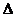PRQ is a right angled triangle, by Pythagoras theorem PQ2 = PR2+RQ2= (x2-x1)2+ (y2-y1)2PQ =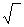{(x2-x1)2+ (y2-y1)2} This  formula is called 'distance formula'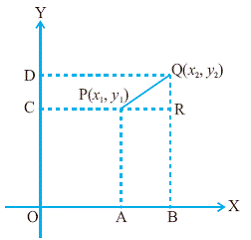Corollary: What if one point is the origin (0,0) ?

The distance of a point P (x,y) from the origin O(0,0)  is  OP =(x2+ y2)

7.2 Problem 1: Find the value of k if P(0,2) is equidistant from Q(3,k) and R(k,5)

Solution:

 PQ ={(3-0)2+ (k-2)2} =(9 +k2-4k+4) PR ={(k-0)2+ (5-2)2} =( k2+9)   Since PQ=PR it follows that 9 +k2-4k+4 = k2+9 On simplification we get k = 1   The point P(0,2) is equidistant from Q(3,1) and R(1,5).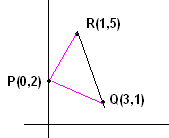7.2 Problem 2: State the special property of the triangle formed by three points A(10,-18), B(3,6) and C(-5,2)

Solution:

 AB ={(3-10)2+ (6-(-18))2} =(49+ 576) =(625) =25 AC ={(-5-10)2+ (2-(-18))2} =(225+ 400) =(625) =25 BC ={(-5-3)2+ (2-6)2} =(64+ 16) =(80)     Since AB=AC it is clear that the triangle formed by the given three points is an isosceles triangle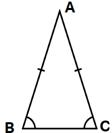7.2 Problem 3: Using distance formula show that points A(2,5), B(-1,2) and C(4,7) are collinear

 Hint: Show that BA+AC = BC (Verify by plotting points that they are collinear)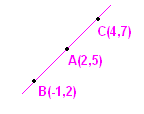7.2 Problem 4:  Find the co-ordinates of the Circumcenter of a triangle ABC whose vertices are A(4,6),B(0,4) and C(6,2)

 Hint: Let O(x,y) be the Circumcenter. Then OA=OB=OC and hence OA2 = OB2 =OC2 Solution: OA2 = (x-4)2+(y-6)2=x2-8x+16+y2-12y+36 OB2 = (x-0)2+(y-4)2=x2+y2-8y+16 OC2 = (x-6)2+(y-2)2=x2-12x+36+y2-4y+4 Equating OA2 = OB2 We get 2x+y =9 Equating OA2 = OC2 We get x-2y = -3 By solving the above two equations we get x=3 and y=3. Hence O(3,3) is the Circumcenter ofABC.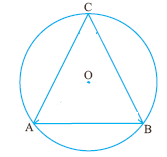7.2.2 Dividing the line in the given ratio:

Section Formula:

This section is about finding a point on a line such that the point divides the line in the given ratio.

 Let AB be the line joining the point A (x1, y1) and B(x2, y2). We are required to find a point P(x, y) on the line AB such that it divides AB in the given ratio of m1:m2. From A, P and B draw perpendiculars to the x-axis and let these perpendiculars meet x- axis at C,Q and D respectively. From A and P draw parallel lines to x axis to meet PQ at E and BD at R. If P is a point on AB dividing it in the given ratio of m1:m2, then AP/PB = m1/m2 It is clear from the adjacent figure that,AEP andPRB are similar (AAA Postulate). Hence AE/PR = PE/BR=AP/PB = m1/m2 --------à(1) AE = OQ-OC = x-x1 : PR = OD-OQ = x2-x PE = QP-QE(=CA) = y-y1 BR = DB-DR = y2-y Thus by substituting values in (1) we get AE/PR = (x-x1)/(x2-x) = PE/PR = (y-y1)/ (y2-y) = m1/m2  --------à(2) By taking first and last expression in (2), we have (x-x1)/(x2-x) = m1/m2m2(x-x1) = m1(x2-x) (By cross multiplication)m2x - m2x1 = m1x2- m1x (By expansion)x(m2+m1) = m1x2+ m2x1(By transposition)x = (m1x2+ m2x1)/(m2+m1)(By division) Similarly, by taking second and last expression in (2) we get  y = (m1y2+ m2y1)/(m2+m1) Thus the co-ordinates of point P, which divides the line joining points A(x1, y1) and B(x2, y2) in the ratio of m1:m2 are given by: {(m1x2+ m2x1)/(m1+m2), (m1y2+ m2y1)/(m1+m2) } This is known as ‘section formula’.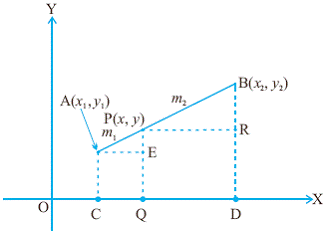1. What are the co-ordinates of midpoint of AB (i.e. when m1:m2 = 1:1)?

It is {(x2+x1)/2), (y2+ y1)/2}:  (Mid point formula)

Note: The above formula can be used to show that the quadrilateral formed by joining the midpoints of adjacent sides of a quadrilateral is a parallelogram.

2. What are the co-ordinates of the point which divides the line in the ratio of k:1?

It is {(kx2+x1)/(k+1), (ky2+ y1)/(k+1)}

7.2 Problem 5:  Find the ratio in which the point P(11,15) divides the line segment joining the points A(15,5) and B(9,20)

Solution:

 Let P divide the line AB in the ratio of k:1 x1=15, y1=5, x2=9, y2=20,x=11, y=15 We have seen above that the co-ordinates of the point which divides a line in the ratio of k:1 is {(kx2+x1)/(k+1), (ky2+ y1)/(k+1)}x = (kx2+x1)/(k+1), y = (ky2+ y1)/(k+1)x = 9k+15/(k+1)11 = 9k+15/(k+1)(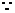it is given that x=11)11k+11 = 9k+152k=4 or k=2 Hence the point divides the given line in the ratio of 2:1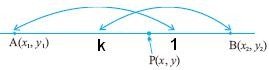7.2 Problem 6:  Find the co-ordinates of points which trisects the line joining A(6,-2) and B(-8,10)

Hint:

 We are required to find the co-ordinates of P and Q such that AP=PQ=QB (1:1:1) This problem needs to be solved in two steps: 1.  Find the co-ordinates of P(x1,y1) such that AP:PB = 1:2. 2.  Find the co-ordinates of Q(x2,y2) such that AQ:QB = 2:1 They are P (4/3,2) and Q (-10/3,6)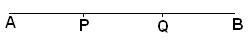7.2 Problem 7:  In triangle ABC, D(-2,5) is mid point of AB, E(2,4) is mid point of BC and F(-1,2) is mid point of AC.

Find the co-ordinates of A,B and C.

 Hint: Let A =(x1,y1), B=(x2,y2) and C=(x3,y3) Since D is mid point of AB, (x1+x2)/2 = -2 and (y1+y2)/2 = 5 Since E is mid point of BC, (x2+x3)/2 = 2 and (y2+y3)/2 = 4 Since F is mid point of AC, (x1+x3)/2 = -1 and (y1+y3)/2 = 2 By solving these three equations we get x1= -5, x2=1, x3= 3 y1= 3, y2=7, y3= 1 Thus the vertices are A(-5,3), B(1,7) and C(3,1)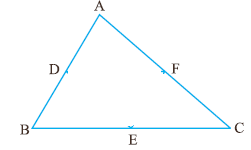7.2 Summary of learning

 No Points to remember 1 The distance between points  P (x1,y1) and Q (x2,y2)  is ={(x2-x1)2+ (y2-y1)2} 2 The  co-ordinates of the point which divides A(x1,y1) and B (x2,y2) in the given ratio of m1:m2  are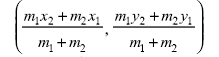Co-ordinates of centroid:

 We have learnt that the centroid divides the median in the ratio of 2:1 (Refer to Section 6.4) Given the three vertices of a triangle let us calculate the co-ordinates of the centroid. In the adjoining figure A (x1, y1), B(x2, y2) and C(x3, y3) AD is one median and G is the centroid which divides AD in the ratio 2:1. We are required to find the co-ordinates of G. Since AD is median BD = DCThe co-ordinates of D are {(x2+x3)/2), (y2+ y3)/2} Since G(x,y) divides AD in 2:1 (m1=2 and m2=1) We have x = {2(x2+x3)/2)+1. x1}/(2+1) = (x2+x3+x1)/3 y = {2(y2+y3)/2)+1. y1}/(2+1) = (y2+y3+y1)/3   Thus the co-ordinates of centroid are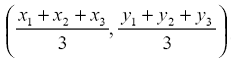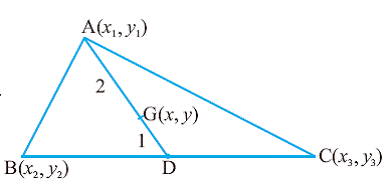7.2 Problem 8: Find the third vertex of a triangle if two of its vertices are at A(-3,1) and B(0,-2) and the centroid is at the origin.

Solution:

Let C(x3, y3) be the third vertex

We know that the co-ordinates of centroid are

x = (x1+x2+x3)/3 and

y = (y1+y2+y3)/3.

Since the co-ordinates of origin are (0,0)

It follows that

x1+x2+x3 = 0  and y1+y2+y3 = 0

By substituting the values for co–ordinates we have

x1+x2+x3= -3+0+ x3=0x3 = 3

y1+y2+y3= 1-2+ y3=0y3 = 1

Thus (3,1) is the third vertex.

Area of a triangle given the co-ordinates of a triangle

 As in the adjoining figure let A (x1, y1), B(x2, y2) and C(x3, y3) be the three vertices of a triangle. We are required to find the area of triangle ABC. Let BL, AM and CN be perpendiculars from the vertices B, A and C to x-axis.OL = x2, OM = x1, ON = x3 and BL = y2, AM = y1, CN = y3   From the figure Area of Triangle ABC = =Area of trapezium BLMA + Area of trapezium AMNC - Area of trapezium BLNC = 1/2(BL+AM)LM + 1/2(AM+NC)MN - 1/2(BL+NC)LN = 1/2(y2+ y1) (x1- x2) + 1/2(y1+ y3) (x3- x1) - 1/2(y2+ y3) (x3- x2) = 1/2[x1(y2- y3) + x2(y3- y1) +x3(y1- y2)] --------- (By rearranging the terms)     Note that if A B and C are collinear then area is zero.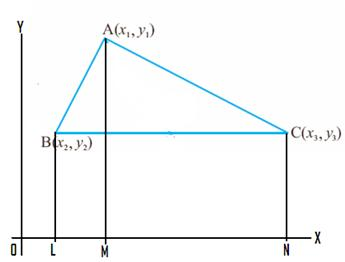7.2 Problem 8: If D(3,-1), E(2,6), F(-5,7) are the mid points of the sides ofABC, find the area ofABC.

 Let us calculate the area ofDEF first Area ofDEF = 1/2[3(6-7)+2(7-(-1))+(-5)(-1-6)] =1/2[-3+16+35] =1/2(48) = 24 sq units   Since the area ofABC is four times the area ofDEF (By BPT theorem – Refer 6.13) Area ofABC = 4*24 = 96 sq units